# DAV Class 7 Maths Chapter 11 Worksheet 1 Solutions

The DAV Maths Class 7 Solutions and DAV Class 7 Maths Chapter 11 Worksheet 1 Solutions of Perimeter and Area offer comprehensive answers to textbook questions.

## DAV Class 7 Maths Ch 11 WS 1 Solutions

Question 1.
A rectangular garden 30 m long and 25 m wide has a path 2.5 m wide running all around inside it. Find the area of the path.
Length of the garden = 30 m
Breadth of the garden = 25 m∴ Area of the garden ABCD = length × breadth
= 30 m × 25 m
= 750 m2
Width of the path = 2.5 m
Length of the inner garden EFGH = 30 – 2 × 2.5
= 30 – 5 = 25 m
Breadth of the inner garden EFGH = 25 – 2 × 2.5
= 25 – 5 = 20 m
Area of the inner garden EFGH = length × breadth
= 25 × 20 = 500 m2
∴ Area of the path = area of ABCD – area of EFGH
= 750m2 – 500 m2
= 250 m2.

Question 2.
A path 1 m wide is built inside a square park of side 30 m along its sides. Find the area of the path. Calculate the cost of constructing the path at the rate of ₹ 70 per m2 .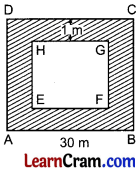Side of the square park = 30 m
∴ its area = (side)2
= (30)2 = 900 m2
Width of the path = 1 m
Length of the side of the inner square = 30 m – 1 × 2 m
= 30m – 2m = 28m
∴ Area of the inner square EFGH = (28)2 = 784 m2
Area of the path = Area of square ABCD – area of square EFGH
= 900 m2 – 784 m2
= 116 m2
Cost of constructing the path = 116 × 70
= ₹ 8120
Hence the required area = 116 m2 and the required cost = ₹ 8120.Question 3.
A table cover 5 m × 3 in, is spread on a meeting table. If 25 cm of the table cover is hanging all around the table, find the area of the table top.
Area of the table cover = 5 m × 3 m = 15 m2
Width of the table cover hanging all around the table
= 25 cm = 0.25 m
Length of the table top = 5 m – 0.25 × 2 m
= 5 m – 0.5 m = 4.5 m
Breadth of the table top = 3 m – 0.25 × 2 m
= 3 m – 0.5 m = 2.5 m
∴ Area of the table top = l × b
= 4.5 × 2.5 = 11.25 m2
Hence the required area = 11.25 m2.

Question 4.
A garden is 120 m long and 85 m wide. It has an inside path of uniform width 3.5 m all around it. The remaining part of the garden is covered by grass. Find the cost of covering the garden by grass at 50 paise per square metre.Length of the garden ABCD
= 120 m
Breadth of the garden = 85 m
Area of the garden ABCD= 120 m × 85 m
= 10200 m2
Width of the path = 3.5 m2
Length of the inner garden EFGH = 120 – 2 × 3.5
= 120 m – 7 m = 113 m
Breadth of the inner garden EFGH = 85 – 2 × 3.5
= 85m – 7m = 78 m
∴ Area of the inner garden EFGH = 113 m × 78 m
= 8814 m2
Cost of. grass to be planted in the inner garden EFGH.
= ₹ 4407
Hence the required cost = ₹ 4407.

Question 5.
Calculate the area of the shaded region in the given figure.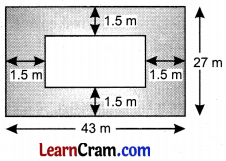Area of the outer rectangle
= l × b
= 43 × 27 = 1161 m2

Length of the inner rectangle
= 43 – 2 × 1.5 = 43 – 3 = 40 m

Breadth of the inner rectangle = 27 – 2 × 1.5
= 27 – 3 = 24 m

∴ Area of the inner rectangle = l × b
= 40 m × 24 m
= 960 m2

Area of the path = Area of the outer rectangle – Area of the inner rectangle
= 1161 m2 – 960 m2
= 201 m2
Hence the required area = 201 m2.

Question 6.
A rectangular lawn measuring 50 m by 36 m is surrounded externally by a path which is 2 m wide. Find the area of the path. Also calculate the cost of levelling of the path at ₹ 16 per m2.Area of the lawn = l × b
= 50 m × 36 m = 1800 m2
Width of the path = 2 m
Length of the external rectangle = 50 + 2 × 2 = 50 + 4 = 54 m
Breadth of the external rectangle = 36 + 2 × 2 = 36 + 4 = 40 m
Area of the external rectangle = 54 × 40 = 2160 m2
Area of the path = 2160 – 1800 = 360 m2
Cost of levelling the path = 360 × 16 = ₹ 5760
Hence the required area = 360 m2 and
the required cost = ₹ 5760.Question 7.
A painting 40 cm long and 28 cm wide is painted on a cardboard such that there is a margin of 2 cm along each of its sides. Find the total area of the margin.
Area of the painting = l × b
= 40 cm × 28 cm = 1120 cm2
Width of the margin = 2 cm
Length of the cardboard = 40 + 2 × 2 = 44 cm
Breadth of the cardboard = 28 + 2 × 2 = 32 cm
Area of the cardboard = 44 × 32 = 1408 cm2
Area of the margin = Area of the cardboard – area of the painting
= 1408 cm2 – 1120 cm2
= 288 cm2
Hence the required area = 288 cm2 .

Question 8.
Each side of a square flower bed is 3 m 80 cm long. It is extended by digging a strip 50 cm wide all around it. Find the area of the enlarged flower bed and also the increase in the area of the flower bed.
Length of the side of square bed = 3 m 80 cm = 3.80 m
Area of this flower bed = (side)2 = (3.80)2
= 14.44 m2
Width of the strip = 50 cm = 0.50 m
Length of square including the strip = 3.80 + 0.50 × 2 = 4.80 m
∴ Area of this extended square = 4.80 m × 4.80 m = 23.04 m2
∴ Increase in area = 23.04 m2 – 14.44 m2 = 8.60 m2
Hence the required extended area = 23.04 m2 and the increase in area = 8.60 m2.### DAV Class 8 Maths Chapter 11 Worksheet 1 Notes

Perimeter:
Total length of the boundary of a closed figure is called its perimeter.

Example:
(i)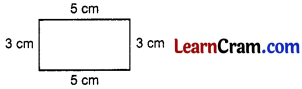Perimeter = 3 cm + 5 cm + 3 cm + 5 cm = 16 cm

(ii)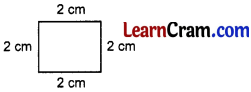Perimeter = 2 × 4 = 8 cm

(iii)Perimeter = 4 cm + 3.5 cm + 2 cm + 3 cm = 12.5 cm

(iv)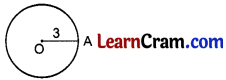Perimeter = 2πrArea:
The magnitude of the plane region is called its area.

Example:

(i)Area = 4 × 3 = 12 cm2

(ii)Area = 3 × 3 = 9 cm2

• Area of a rectangular path inside (or outside) a rectangular field = Area of the outer rectangle – Area of the inner rectangle
• Area of cross paths = Area of all the rectangles making the paths – Area of the common rectangle (or square)
• Area of a parallelogram = Base × Height
• Area of a triangle = $$\frac{1}{2}$$ × Base × Height
• Area of an equilateral triangle = $$\frac{\sqrt{3}}{4}$$ × (side)2
• Area of a rhombus = 4d1 × d2, where d1 and d2 are the diagonals of the rhombus.
• The perimeter of a circle is called its circumference.
The constant ratio of the circumference of a circle to its diameter is denoted by πr.
• Circumference of a circle = 2π × Radius of circle
Area of a circle = π × (radius)2Example 1.
A rectangular park is 30 m wide and 40 m long. 5 m wide walking track is made to run round inside the park. Find the area of the track.Length of the park = 40 m
Breadth of the park = 30 m
Area of the park = 40 × 30 = 1200 m2
Width of the track = 5 cm.
Length of the inner rectangle = 40 – 5 × 2
= 40 – 10 = 30 m
Breadth of the inner rectangle = 30 = 5 × 2 m
= 30 – 10 = 20 m
∴ Area of the inner rectangle = 30 × 20 = 600 m2
Area of the track = Area of the bigger rectangle – Area of the smaller rectangle
= 1200 m2 – 600 m2 = 600 m2.

Example 2:
A rectangular garden is 40 m by 25 m. It has 2.5 m wide two roads running in the middle of it, one is parallel to length and other is parallel to its breadth. Find the area of the roads and the area of the remaining garden.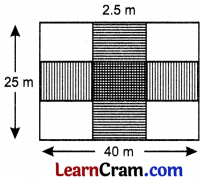Area of the garden = length × breadth
= 40 × 25
= 1000 m2
Area of the road parallel to the length = 40 × 2.5 = 100 m2
Area of the road parallel to the breadth = 25 × 2.5 = 62.5m2
Area of the common region = 2.5 × 2.5 = 6.25 m2
∴ Area of the two roads = 100 m2 + 62.5 m2 – 6.25 m2
= 162.5 m2 – 6.25 m2
= 155.25 m2
Area of the remaining garden = 1000 m2 – 155.25 m2 = 844.75 m2Example 3:
Find the area of a rhombus whose each side is 4.5 cm and the D c altitude is 3.2 cm.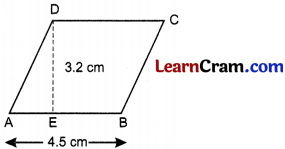Area of the rhombus = base × altitude
= 4.5 × 3.2
= 14.40 cm2

Example 4:
If the side of an equilateral triangle is 4.8 cm, find its area.
Area of equilateral ∆ = $$\frac{\sqrt{3}}{4}$$ × (side)2
= $$\frac{\sqrt{3}}{4}$$ × (4.8)2
= $$\frac{\sqrt{3}}{4}$$ × 4.8 × 4.8
= $$\frac{\sqrt{3}}{4}$$ × 1.2 × 4.8
= 5.76√3 cm2Example 5:
The length of parallel sides of a trapezium are 3.2 cm and 4.8 cm, the distance between the parallel sides is 3.6 cm. Find the area.Area of trapezium = $$\frac{1}{2}$$ × (sum of parallel sides) × altitude
= $$\frac{1}{2}$$ × (AB + DC) × DE
= $$\frac{1}{2}$$ × (4.8 + 3.2) × 3.6
= $$\frac{1}{2}$$ × 8 × 3.6
= $$\frac{1}{2}$$ × 3.6
= 14.4 cm2
Hence the area of the trapezium 14.4 cm2.

Example 6:
Find the area of a rhombus whose diagonals are 6 cm and 4 cm.
Area of rhombus = 4 × Product of the diagonals
= $$\frac{1}{2}$$ × 6 × 4
= 3 × 4
= 12 cm2.

Example 7:
In a right triangle ABC, ∠B = 90°, hypotenuse AC = 29 cm. If one side BC = 20 cm, find its area.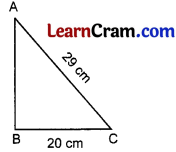In right triangle ABC,
AB2 + BC2 = AC2
AB2 + (20)2 = (29)2
AB2 + 400 = 841
AB2 = 841 – 400
AB2 = 441
∴ AB = $$\sqrt{441}$$ = 21 cm
Area of ∆ ABC = $$\frac{1}{2}$$ × 20 × 21
= $$\frac{1}{2}$$ × 20 × 21
= 10 × 21
= 210 cm2Example 8:
In the given figure, find the area of a □ ABCD.Area of Δ ABD = $$\frac{1}{2}$$ × 10 × 4
= 5 × 4
= 20 cm2
Area of Δ BCD = $$\frac{1}{2}$$ × 10 × 6
= 5 × 6
= 30 cm2
Area of □ ABCD = area of Δ ABD + area of Δ BCD
= 20 cm2 + 30 cm2 = 50 cm2

Example 9:
A wire of length 88 cm is to be bent into a circle. Find the area of the circle so formed.
Circumference of the circle = 88 cm
∴ 2πr = 88 cm
2 × $$\frac{22}{7}$$ × r = 88
r = $$\frac{88 \times 7}{2 \times 22}$$
= 14 cm
∴ Area of the circle = πr2
= $$\frac{22}{7}$$ × 14 × 14
= 22 × 2 × 14
= 616 cm2.Example 10.
The diameter of a circular park is 28 m. A 2.1 m wide path runs around it from inside. Find the cost of paving the path at ₹ 2 per sq. m.
The diameter of the circular park = 28 m
Its radius = $$\frac{28}{2}$$ = 14 m
∴ Area of the park = πr2
= π × (14)2 m2
Width of the circular path = 2.1 m
∴ Radius of the inner circle = 14 m – 2.1 m = 11.9 m
∴ Area of the inner park = π (11.9)2 m2
∴ Area of the path = π × (14)2 – π × (11 .9)2
= π [(14)2 – (11.9)2]
= π × (14 + 11.9) (14 – 11.9)
= $$\frac{22}{7}$$ × 25.9 × 2.1
= 22 × 25.9 × 0.3
= 170.94 m2
Hence the required area = 170.94 m2
and the required cost for paving the path = 170.94 × 2 = ₹ 341.88.